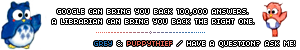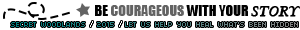(See All?) Announcements
48 Users Online
Bing

Print · · Subscribe · 0 Loves ·
Askan is attacking in his next post.

Stats are as follows:

HP - 47
DEF - 20
ATK - 13
DMG - 14
IN - 2

Lorcan:
HP - 34
DEF - 15
ATK - 12
DMG - 9
IN -4

@Lorcan , plz consent. And may we have it all rolled out at once <3I consent >:D
# P O R C A N
sometimes, two people have to fall apart to realise
how much they need to fall back together
Code:
`[attacker="Askan" HP="47" DEF="20" ATK="13" DMG="14" IN="2"][defender="Lorcan" HP="34" DEF="15" ATK="12" DMG="9" IN="4"]`

Roll Results:
Askan rolls a d15 and gets a 7.
13 + 7 = 20

Lorcan's DEF: 15
20 > 15

The attack was a HIT!

Askan rolls a d6 and gets a 6.
14 + 6 = 20

Lorcan's HP: 34
34 - 20 = 14

Lorcan's HP: 14PLOT WITH ME!
Code:
`[attacker="Lorcan" HP="14" DEF="15" ATK="12" DMG="9" IN="4"][defender="Askan" HP="47" DEF="20" ATK="13" DMG="14" IN="2"]`

Roll Results:
Lorcan rolls a d15 and gets a 9.
Lorcan's' ATK: 12
12 + 9 = 21

21 > 20

The attack was a HIT!

Lorcan rolls a d6 and gets a 4.
Lorcan's DMG: 9
9 + 4 = 13

47 - 13 = 34

Lorcan's HP: 14PLOT WITH ME!
Code:
`[attacker="Askan" HP="34" DEF="20" ATK="13" DMG="14" IN="2"][defender="Lorcan" HP="14" DEF="15" ATK="12" DMG="9" IN="4"]`

Roll Results:
Askan rolls a d15 and gets a 13.
13 + 13 = 26

Lorcan's DEF: 15
26 > 15

The attack was a HIT!

Askan rolls a d6 and gets a 4.
14 + 4 = 18

Lorcan's HP: 14
14 - 18 = -4

Lorcan's HP: -4